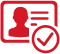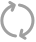（安卓/ios）(安卓/iOS)载入中...

• 安卓
• 苹果载入中...
选择区服
搜索载入中...
已选条件：
账号
商品类型:
系统:
客户端:
价格区间:
身份证:
手机:
邮箱:
高级筛选:
• 热门枪械
• AUG妙趣可丽
• AUG星之彼岸
• AKM星际浪客
• AKM甜心宝贝
• AKM炫紫旋律
• AKM梦之光华
• AKM百合蜜语
• AWM橘柚倾心
• AWM焰龙焚天
• AWM花间之火
• AWM平天焚空
• AKM盖世英雄
• DP28扶摇飞仙
• DP28枪炮玫瑰
• DP28幻梦音符
• DP28暗夜鎏金
• GROZA香水柠檬
• GROZA旋转彩虹
• GROZA焦糖拿铁
• Kar98K暗翼战神
• Kar98K绿意幻情
• Kar98K伏魔团
• Kar98K圣光天使
• MG3洛神天依
• MG3炙焰牛王
• M24暗星紫秘
• M24星之信仰
• M24翩跹演武
• M416五爪金龙
• M416心之恋语
• M416风花雪月
• M416暖熊萌萌
• M416至情至圣
• M762甜心娃娃
• M762激战未来
• M762甜蜜誓约
• M762冷魅冰骑
• M762苔丝玫瑰
• M249彩虹甜喵
• M249古战神甲
• M249蓝莓炮弹
• M16A4葫芦兄弟
• M16A4垂柳戏鸭
• Mini14龙虾霸霸
• Mini14荧光虫舞
• Mini14绚金瑰梦
• MK20H海螺星光
• SKS诡面斩
• SKS碧影闪光
• SLR暗夜玫瑰
• S12K海渊国主
• SCARL天蓬元帅
• SCARL纯梦嫁纱
• SCARL桃夭倾城
• SCARL机甲封神
• SCARL童趣迷兔
• SCARL梦幻火箭
• SCARL青春物语
• SCARL甜梦小狐
• UZI绮梦青鸾
• UZI筑梦精灵
• UMP45启明星
• UMP45相思残雪
• UMP45雪国精灵
• UMP45恐龙拽拽
• UMP45玉魂晶魄
• VSS烈焰拳霸
• Vector红伞翩翩
• Vector环太平洋
• Vector灵感创作师
• 野牛流星咚咚
• 汤姆逊怪笑疯兔
• 汤姆逊荒漠赏金
• 汤姆逊冰川粉黛
• 平底锅林间仙鹿
• 平底锅仲夏莫吉托
• UZI畅跳缤纷
• DP28金蝉行者
• SPAS12音爆索尼克
• 野牛青空秋雨
• P90甜蜜草莓
• M249绒雪飘舞
• SCARL镭射皇者
• 爆炸猎弓雪梅暗香
• M417第五大道
• M416全功率超战
• Vector飒粉甜心
清空确定
• 稀有套装
• 洛天依
• 向日葵
• 诡面斩
• 木乃伊
• 启明星
• 闪光银龙
• 烈焰拳霸
• 狩月元帅
• 旋转彩虹
• 金风银杏
• 黄伞悠悠
• 暗翼战神
• 深海曼妙
• 土曜战麒
• 未来战士
• 暖熊萌萌
• 碧穹智者
• 霁雪冰心
• 彗云及圣
• 霞光圣骑
• 冷魅冰骑
• 寒光使者
• 天之羽翼
• 酣梦娃娃
• 翡翠骑士
• 甜蜜誓约
• 琉璃脉冲
• 纯珍童话
• 心之回忆
• 挚爱序曲
• 海螺星光
• 幻情紫藤
• 薄荷物语
• 甜心娃娃
• 骨龙祭渊
• 枪炮玫瑰
• 绿野柔风
• 童趣迷兔
• 金风玉露
• 紫电风暴
• 赤焰黑爵
• 暗夜鎏金
• 垂柳戏鸭
• 碧影闪光
• 桀骜红莲
• 蝶舞蔷薇
• 相思残雪
• 星之彼岸
• 锦绣火鲤
• 新星烈龙
• 天启纪元
• 霞光骑士
• 甜蜜草莓
• 花影阑珊
• 梦幻火箭
• 闪耀迪迦
• 荒漠赏金
• 桃夭倾城
• 冰川粉黛
• 冰川幽秘
• 冰川红玫
• 彩虹甜喵
• 霓裳武神
• 焦糖拿铁
• 暗星紫秘
• 精灵娃娃
• 流星咚咚
• 绚金瑰梦
• 青葱仙萝
• 蓝莓炮弹
• 红伞翩翩
• 黑伞漫漫
• 海渊国主
• 齐天大圣
• 沙场疾风
• 俏雪含霜
• 吟月归客
• 林间仙鹿
• 彩虹鹿灵
• 山涧鹿灵
• 暗夜玫瑰
• 炙焰赤龙
• 潮汐统领
• 花间之火
• 扶摇飞仙
• 心之恋语
• 极致幻想
• 星空之魅
• 慧云及圣
• 天禅智恒
• 古堡领主
• 金属狂野
• 静灵氛围
• 智能音符
• 缤纷油彩
• 放客神游
• 华贵君王
• 星之信仰
• 精灵迷巫
• 蝶舞迷情
• 雪国幻梦
• 守护骑士
• 银河战甲
• 金曜白虎
• 木曜青龙
• 水曜玄武
• 火曜朱雀
• 土曜战麟
• 深渊皇后
• 荒野猎神
• 粉色回忆
• 南瓜骑士
• 数码大师
• 幻影战神
• 极限特训
• 快乐主宰
• 独舞天鹅
• 海盐物语
• 怪诞乔克
• 岚岚物语
• 百合蜜语
• 恐龙拽拽
• 紫鸢之舞
• 星云玉兔
• 雪国梦幻
• 折纸暴龙
• 缤纷油菜
• 浪漫波比
• 黄金大亨
• 天使彩虹糖
• 灵感创作师
• 王牌四分卫
• 幻彩马卡龙
• 麻辣鲜香锅
• 甜心巧克力
• 宝贝奶油派
• 星际漫游者
• 赛罗闪耀型
• 星夜魔法师
• 复仇流浪者
• 专家巡查员
• 深海小丑鱼
• 奇异狩猎者
• 闪耀奥特曼
• 101粉红套装
• 至尊金龙套装
• 黑曜金尊套装
• 2号机款机体服
• PEL轰鸣竞梦者
• 初号机款机体服
• 女娲后裔赵灵儿
• 蜀山剑侠李逍遥
清空确定
• 稀有飞行器
• 阿狸红
• 诡面斩
• 马卡龙
• 伏魔团
• 甜心娃娃
• 机甲封神
• 纯梦嫁纱
• 闪耀银龙
• 庄周梦蝶
• 春暖花开
• 桃天倾城
• 冰川粉黛
• 绒雪飘舞
• 闪光银龙
• 霓虹街区
• 甜梦小狐
• 幻梦音符
• 冷魅冰骑
• 青春物语
• 焦糖拿铁
• 天之羽翼
• 绚金瑰梦
• 绿意幻情
• 红伞翩翩
• 齐天大圣
• 风花雪月
• 林间仙鹿
• 焰龙焚天
• 枪炮玫瑰
• 古战神甲
• 梦之光华
• 相思残雪
• 星之彼岸
• 绮梦青鸾
• 暗翼战神
• 终极赛罗
• 童趣迷兔
• 激战未来
• 锦绣火鲤
• 潮汐统领
• 花间之火
• 扶摇飞仙
• 心之恋语
• 四圣焕新
• 甜蜜誓约
• 古堡领主
• 神秘黑猫
• 深海曼妙
• 炫紫旋律
• 精灵迷巫
• 梦幻火箭
• 荧光虫舞
• 翩跹演武
• 太空嘟嘟
• 歌者泠鸢
• 苔丝玫瑰
• 星夜魔法师
• 王牌四分卫
• 夏日绵绵冰
• 灵感创作师
• PEL轰鸣竞梦者
• 暗夜鎏金降落伞
• 至尊龙雀飞行服
• 荒漠赏金飞行翼
• 星之信仰飞行翼
• 繁花小夜曲飞行翼
清空确定
• 稀有载具
• 僚机
• 萌兔起飞
• 南瓜骑士
• 焰龙咆哮
• 金龙耀世
• 海龙奔腾
• 萌喵橘座
• 灵鼠银迹
• 鬼马风驰
• 极速兔兔车
• 俏皮小熊猫
• 软萌羊咩咩
• 拉轰鸭鸭车
• 空客H135粉
• 空客H135蓝
• 合金龙骨(蓝)
• 合金龙骨(绿)
• 合金龙骨(红)
• 阿斯顿马丁DBS(金)
• 阿斯顿马丁DBS(红)
• 阿斯顿马丁DBS(绿)
• 阿斯顿马丁DBX(金)
• 阿斯顿马丁DBX(橙)
• 阿斯顿马丁DBX(蓝)
• 迈凯伦570S(镭射紫)
• 迈凯伦570S(炫冰蓝)
• 迈凯伦570S(旭日黄)
• 迈凯伦570S(天际蓝)
• 双人摩托_梦幻火箭
• 双人摩托_双刃魅影（绿）
• 双人摩托_双刃魅影（红）
• 双人摩托_双刃魅影（金）
• 快艇_自由的鲸
• 快艇_塞莎KL27(鲸之蓝)
• 快艇_塞莎KL27(霓虹粉)
• 快艇_塞莎KL27(深潜蓝)
• 路特斯ELETRE(冰川蓝）
• 路特斯ELETRE(电光紫）
• 路特斯ELETRE(花绽灰）
• 路特斯EVJIA(电光紫）
• 路特斯EVJIA(星际黄）
• 特斯拉Model3（奇幻紫）
• 特斯拉Model3（珍珠白）
• 特斯拉Model3（冷光银）
• 特斯拉ModelX（奇幻紫）
• 特斯拉ModelX（冷光银）
• 特斯拉ModelX（珍珠白）
• 特斯拉Cybertruck(镭光铂）
• 特斯拉Cybertruck(荒芜蓝）
• 特斯拉Cybertruck(极昼黑)
• 玛莎拉蒂Ghibli(金）
• 玛莎拉蒂Ghibli(蓝)
• 玛莎拉蒂Ghibli(粉)
• 玛莎拉蒂MC20(幻星粉）
• 玛莎拉蒂MC20(星石白)
• 玛莎拉蒂Grecale(幻星粉)
• 玛莎拉蒂Grecale(薄荷绿)
• 玛莎拉蒂Quattroporte（幻星粉）
• 玛莎拉蒂Quattroporte（夜幕蓝）
• 兰博基尼URUS（明艳黄）
• 兰博基尼URUS(炫光金）
• 兰博基尼URUS(尊享铂）
• 兰博基尼Estoque(炫光金）
• 兰博基尼Estoque(尊享铂)
• 兰博基尼Estoque(银翼灰)
• 乔治巴顿战车（乳石白）
清空确定
防沉迷:
只显示：
温馨提示：

商品说明：此类商品是指游戏账号被卖家身份证绑定，存在账号被找回风险，买家购买需谨慎！

关键词搜索:
• 商品标题
• 价格
• 库存
• 安全保障

# 实名认证根据《中华人民共和国网络安全法》、《中华人民共和国未成年人保护法》等相关法律、法规要求，网络交易需实名制认证，未成年人不得参与网络游戏相关交易行为。为保障您的交易行为安全、合法，请完成实名认证在继续交易。
真实姓名：
身份证号：

# 实名认证# 恭喜实名认证通过！

请继续您的交易请输入正确手机号验证码错误请重新输入
发送下载链接扫码立即下载APP